# Catch up on the latest AI articles# I-BERT: BERT That Can Be Reasoned With Integer Types Only

3 main points
✔️ Proposed I-BERT with all BERT calculations in integer type
✔️ Introduced a new method to approximate Softmax and GELU with quadratic polynomials
✔️ 2.4~4.0 times faster than without quantization

written by Sehoon KimAmir GholamiZhewei YaoMichael W. MahoneyKurt Keutzer
Submitted on 5 Jan 2021 (v1), last revised 8 Jun 2021 (this version, v3)

Subjects: Computation and Language (cs.CL)code：

The images used in this article are from the paper, the introductory slides, or were created based on them.

## Introduction

Transformer-based models such as BERT and RoBERTa have shown high accuracy in many natural language processing tasks. However, memory footprint, inference time, and power consumption pose challenges not only at the edge, but also in data centers.

One method to solve this problem is model quantization, which lightens the weight of the model by expressing model weights and liveness in low-bit precision, such as 8-bit integers (INT8) instead of 32-bit floating-point numbers (FP32).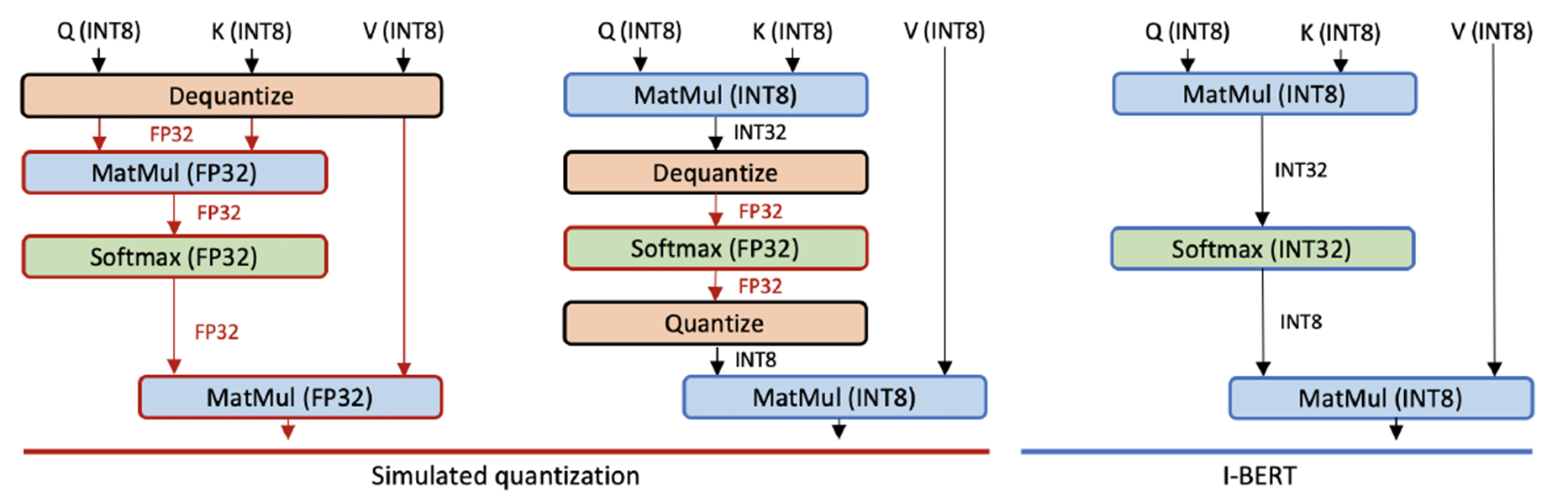The model quantization that has been done so far for Transformer-based models is limited to the input and linear arithmetic part of the model, and it cannot be said that complete model quantization has been done (Simulated quantization shown above).

Therefore, this paper proposes I-BERT, in which all operations in BERT are realized in integer type.

## Proposed method: I-BERT

### Basic Quantization Method

Floating-point to integer quantization is performed using symmetric uniform quantization as follows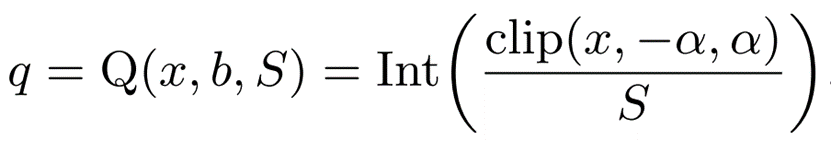x is the floating-point number before quantization and q is the integer value after quantization.

Non-uniform quantization, which dynamically changes the range of floating-point numbers (x) corresponding to q, has the potential to capture the distribution of weights and activation parameters. However, it causes overhead, so uniform quantization is used.

b is bit precision, which determines the range of values that q can represent as followsclip is the truncation function and α is a parameter that controls the range. The reason this method is called symmetric is that the clip truncates the value [-α,α] symmetrically.

S is a scaling factor; S is fixed at inference time as follows to avoid runtime overhead (static quantization)### Non-linear Funcitons with Integer-only Arithmetic

In prior research, linear and piecewise linear operations can be easily performed, since integer-only operations take advantage of linearity. For example, MatMul (matrix product) can be computed by multiplying the quantized value q by a scaling factor S, as in the following equation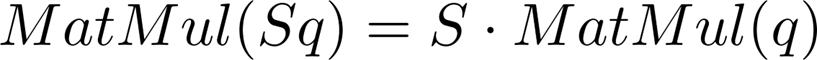On the other hand, nonlinear functions such as GELU cannot be easily calculated because they do not satisfy linearity, as shown in the following equation.To address this issue, this paper proposes approximating nonlinear functions to polynomial functions that can be computed using only integer arithmetic.

### Polynominal Approximation of Non-linear Functions

Interpolating polynominals are used to approximate nonlinear functions to polynomial functions.

Find a polynomial up to degree n that fits the data set of the function at n+1 points, as in the following equation.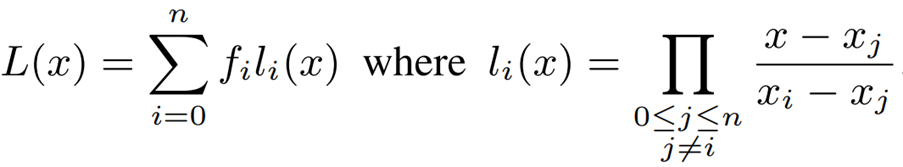Here, the key point is the choice of order n. The larger the order, the smaller the approximation error. The larger the order, the smaller the approximation error, but it also causes issues such as "overhead and increased computation volume" and "possible overflow when computing with low operation bits.

Given the above, the challenge is to find low-degree polynomials that can approximate the nonlinear functions (GELU and Softmax) used in the Transformer. In the following, we will take a closer look at the GELU and Softmax approximation methods proposed in this paper.

### Integer-only GELU

GELU is the activation function used in the TRANSFORMER model and can be expressed as follows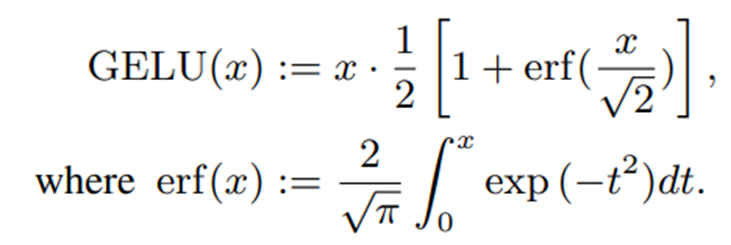Because the integral term of erf (error function) is computationally inefficient, various approximation methods have been proposed.

For example, the following approximations using sigmoid functions are available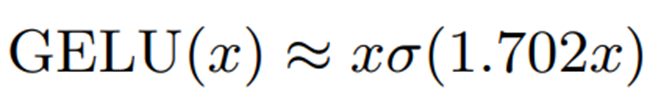The sigmoid function (σ) itself is a nonlinear function and therefore not a suitable approximation method for integer arithmetic.

Another method is to approximate the sigmoid function by hard Sigmoid (h-Sigmoid). In this paper, this approximation method is called h-GELU.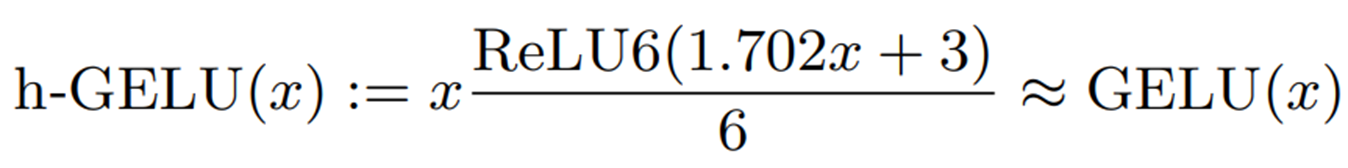Although h-GELU can perform operations only on integer types, there is a problem that reduces the accuracy of the approximation.

Therefore, this paper proposes to approximate the error function by a two-dimensional polynomial.

For approximation, consider the following optimization problem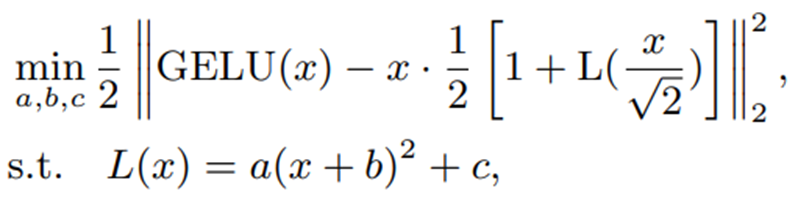L(x) represents the quadratic polynomial that approximates the error function. If the optimization is performed with the entire domain of definition as real numbers, the approximation accuracy will be reduced.

To solve this problem, we consider a limited range approximation, taking advantage of the fact that the error function approaches 1 when x is large.Also, since the error function is an odd function, only positive regions are considered.

Under these conditions, the approximation using interpolating polynominals explained above is as follows.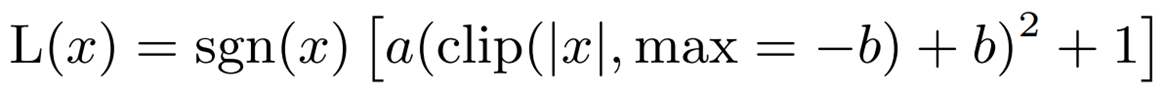a = -0.2888 and b = -1.769, where sgn is the sign function. An approximation of GELU using this polynomial ( i-GELU) can be expressed as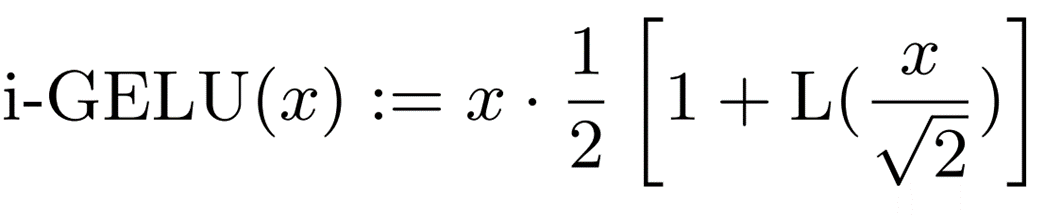The algorithm used by i-GELU for the calculation is as follows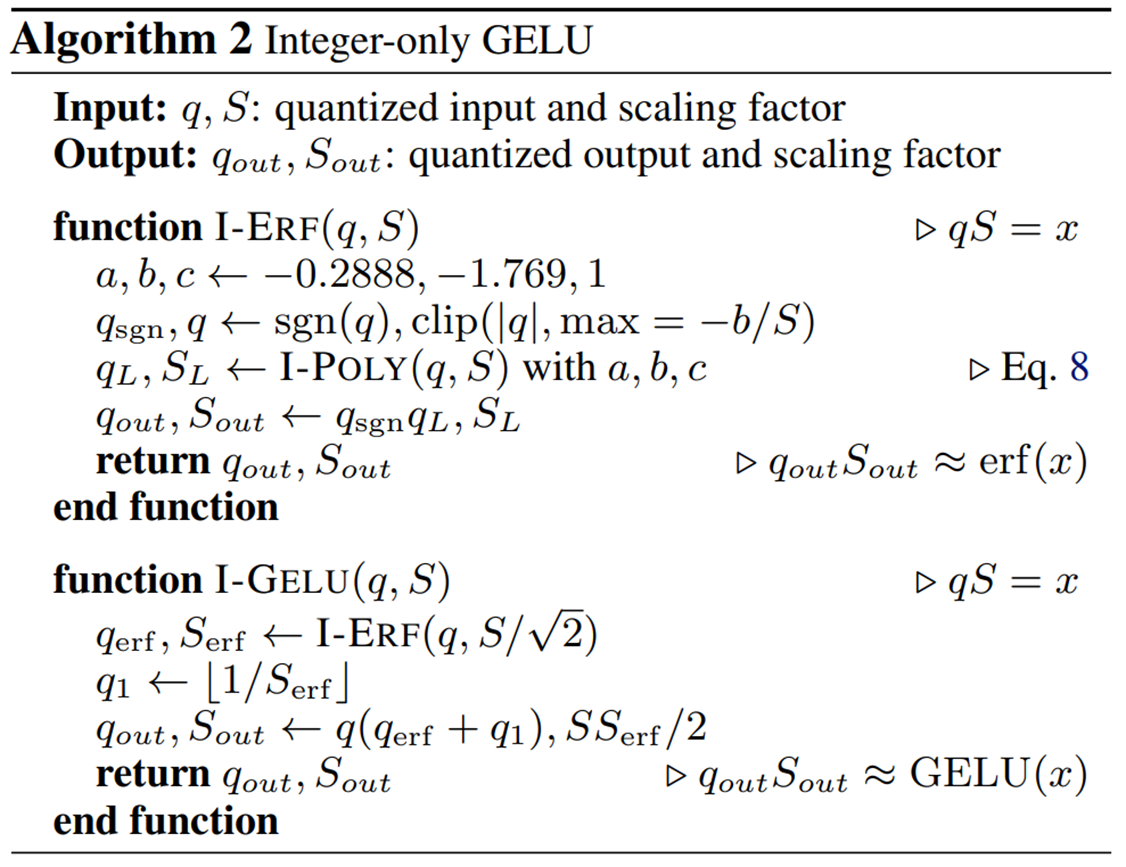The following graph compares i-GELU with the existing method using the sigmoid function, h-GELU.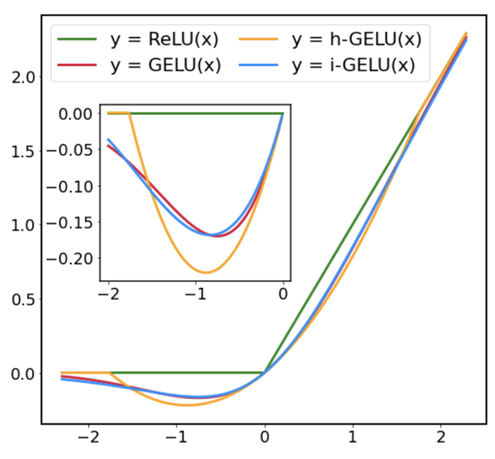The following table shows the results of a quantitative comparison in terms of approximation accuracy (L2 and L∞ distances). Both the graph and the table show that i-GELU has the best approximation accuracy.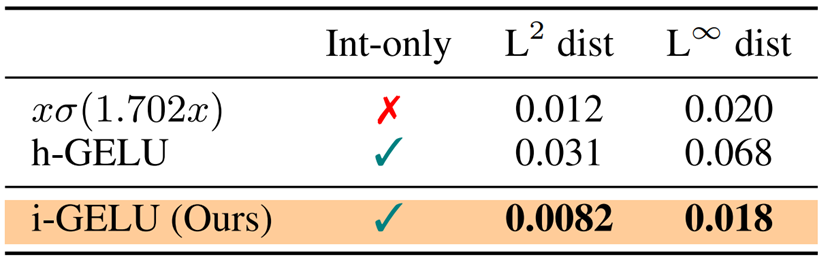### Integer-only Softmax

Softmax is represented by the following equation and can convert an input vector into a probability distribution.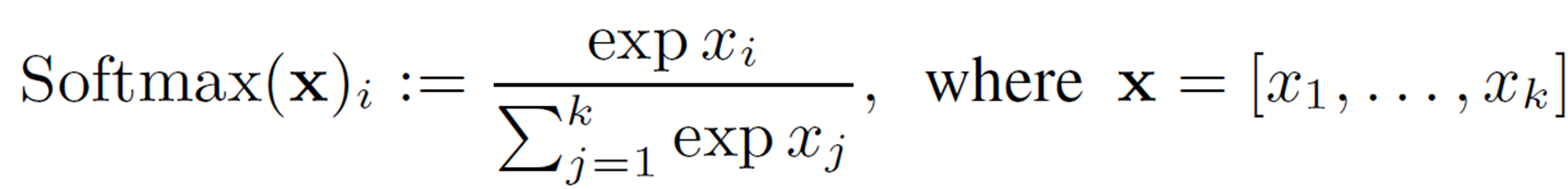Similar to GELU, we consider a quadratic polynomial approximation with a restricted range. First, for numerical stability (to prevent overflow), we subtract the value of the input by the maximum value ().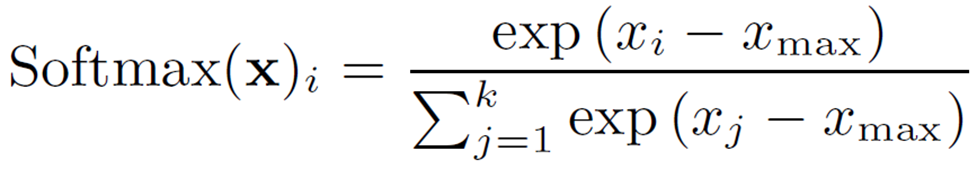Here,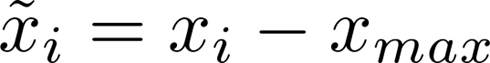is broken down as follows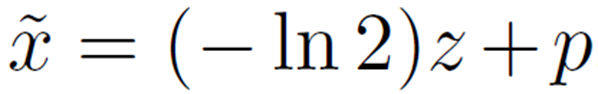z and p represent the quotient and the remainder when divided by -ln2, respectively. The exponential part of Softmax can then be expressed as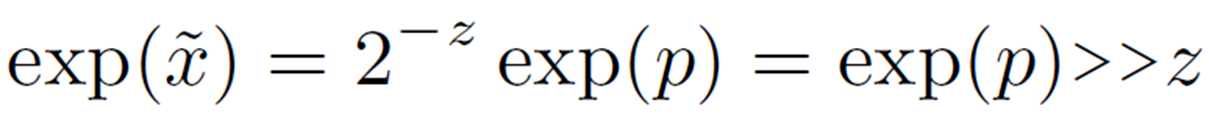Therefore,we consider approximating exp(p) at. As in the case of GELU, applying the interpolating polynominals described above, we can approximate as follows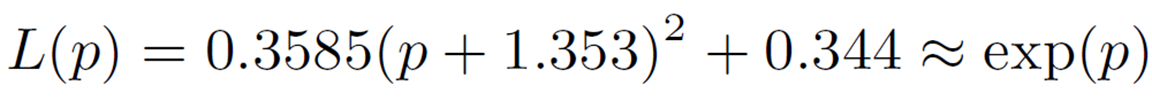Thus,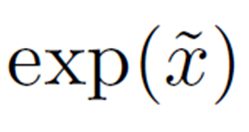can be calculated as follows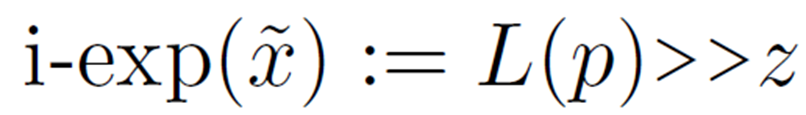Here is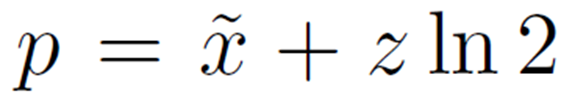,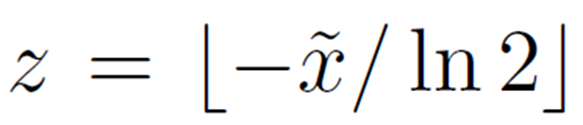. A graphical confirmation of this exponential approximation is shown below.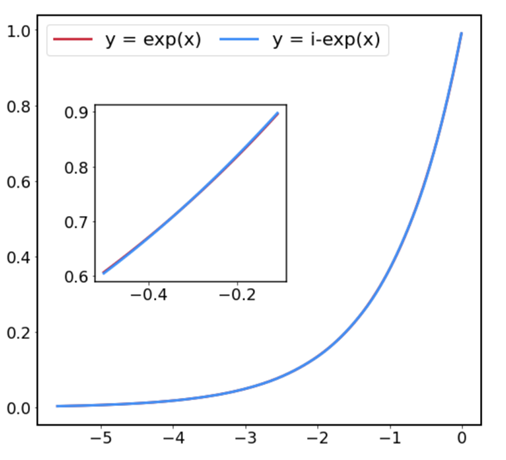The largest error is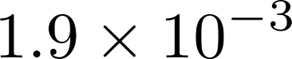, which shows that the error is small enough given that the 8-bit quantization error is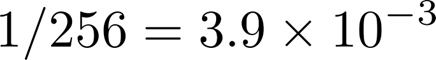. Finally, to summarize up to this point, here is the algorithm to calculate Softmax in integer type.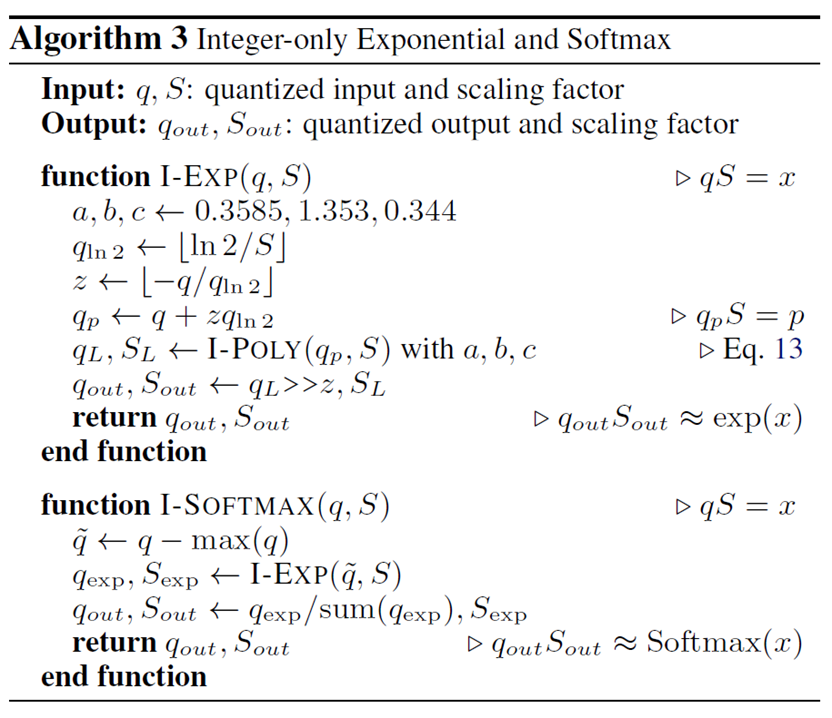### Integer-only LayerNorm

LayerNorm standardizes the input features across channel dimensions and is represented by the following equation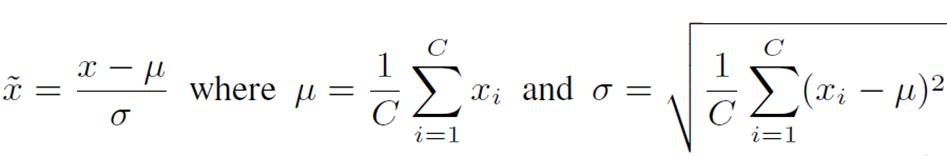μ andσ represent the mean and standard deviation, respectively.

The square root operation required in computing the standard deviation is difficult to compute directly in integer type. Therefore, they are calculated using the iteration algorithm based on Newton's method proposed in .

The algorithm is as follows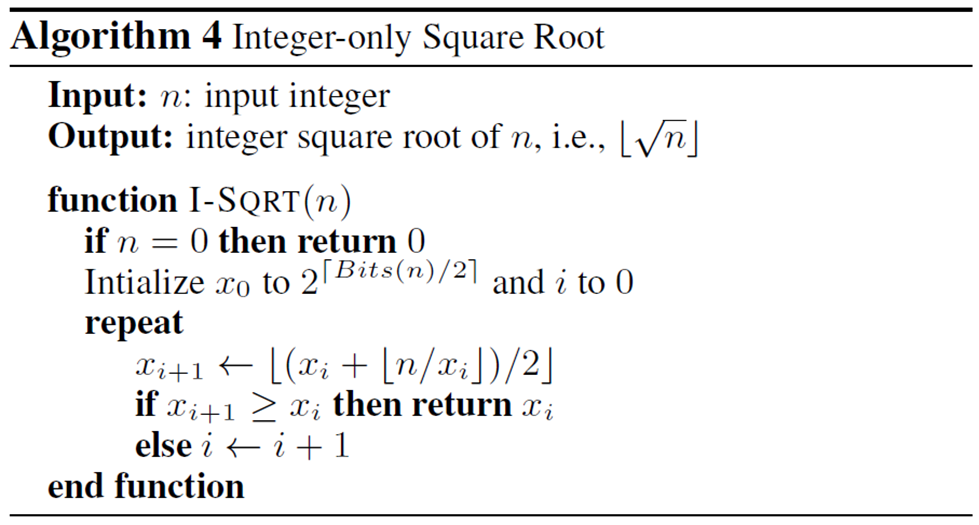## Experiment

### Precision Evaluation

We start by looking at the inference accuracy of I-BERT, which is validated on the RoBERTa-Base and RoBERTa-Large models in a benchmark task called GLUE.

The results are shown in the table below.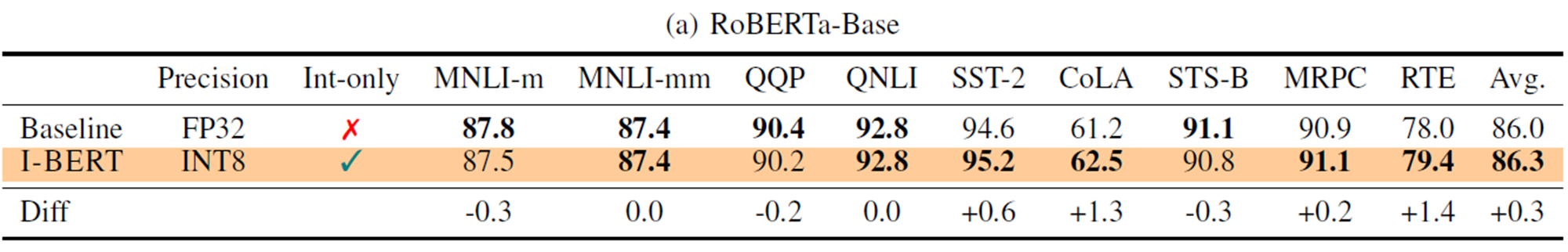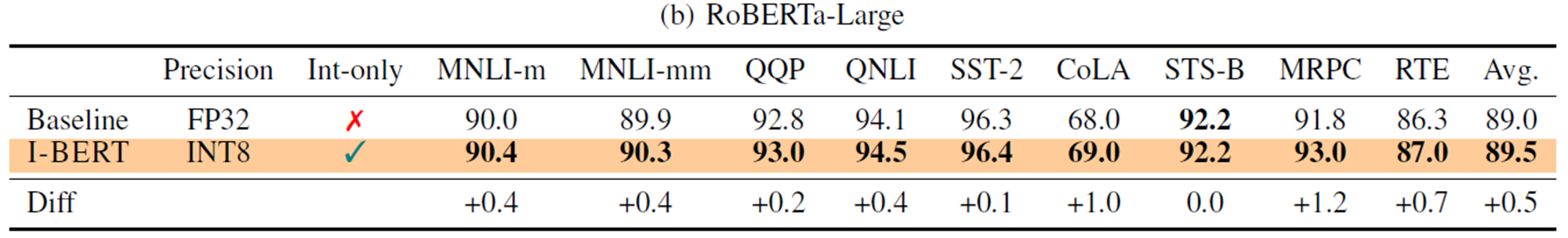RoBERTa-Base showed higher accuracy than when calculated with FP32 (floating point numbers) for all but the MNLI-m, QQP, and STS-B tasks.

Even for those that did lose accuracy, the difference was very small, up to 0.3%, with an average improvement of 0.3%. For RoBERT-Large, I-BERT outperforms I-BERT in all tasks, with an average improvement in accuracy of 0.5%.

In sum, it can be seen that I-BERT achieves similar or slightly higher accuracy than FP32.

### Reasoning speed evaluation

I-BERT's inference speed is also being verified.

The table below shows the speedup compared to FP32 for each sentence length (SL) and batch size (BS). The models used are BERT-Base and BERT-Large.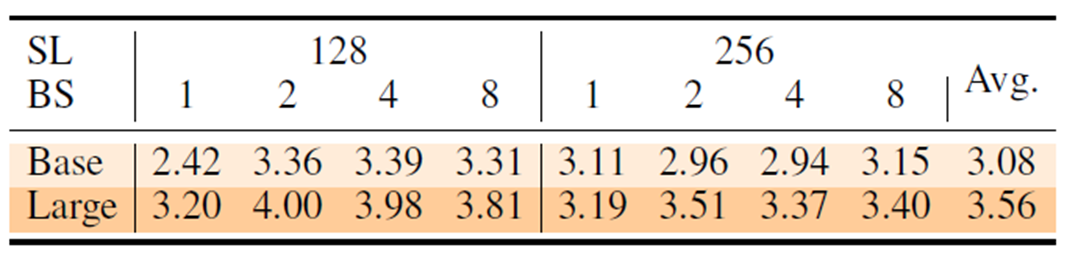We can see that I-BERT, which performs INT8-type inference, is on average 3.08 times faster for BERT-Base and 3.56 times faster for BERT-Large than when inference is performed with FP32.

## Ablation Study

We are also validating i-GELU, the GELU approximation method employed by I-BERT.

In the RoBERa-Large Model, the following table compares GELU without approximation, h-GELU, and i-GELU on the same GLUE task as during the accuracy evaluation .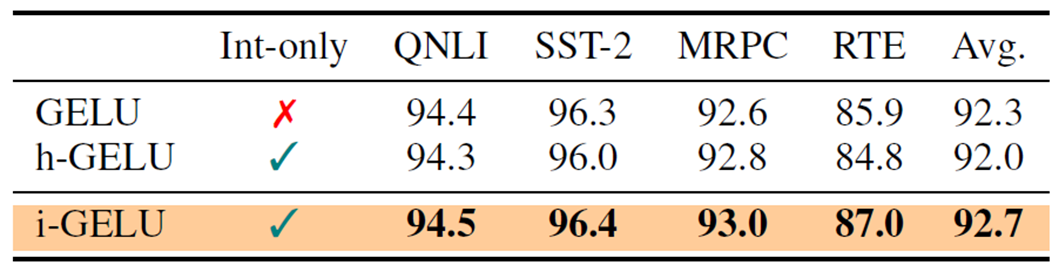It can be observed that h-GELU has lower accuracy than GELU in all tasks except MRPC.This indicates that the approximation accuracy of h-GELU is not sufficient.

The i-GELU outperforms the h-GELU in all tasks and shows equal or slightly better accuracy than the GELU. These results indicate that i-GELU's approximation is adequate.

## Summary

In this issue, we introduced I-BERT, a BERT in which all calculations are performed with integer types.The fact that it achieves a speedup of about 3 times without significant loss of accuracy is a major attraction.

In cases where information that needs to be kept confidential is to be processed by the natural language model at hand, we felt that there may be demand for a model like I-BERT that is light on computational processing.

## Supplement

 Verified on Tesla T4 GPUs.

If you have any suggestions for improvement of the content of the article,